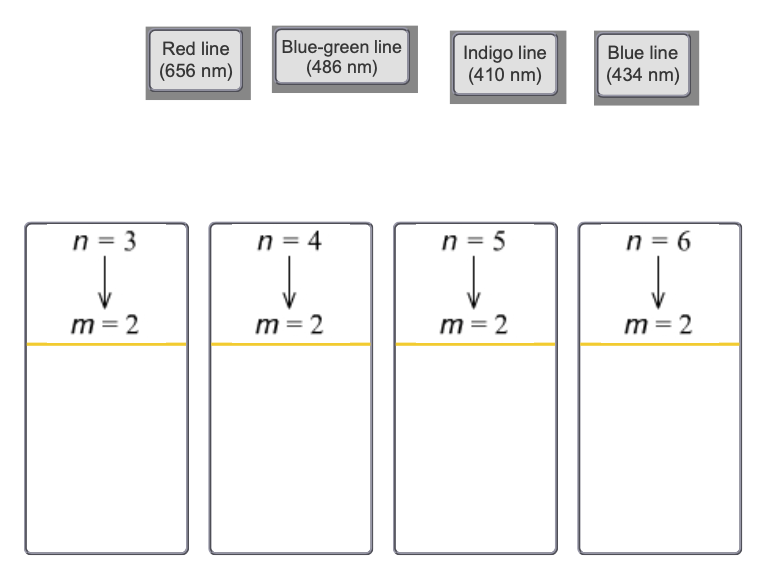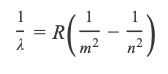# Problem: Part B. The image shows the wavelengths (in nanometers) of the four visible lines in the Balmer series for hydrogen. Match each line to its corresponding transition.Drag each item to the appropriate bin.Atoms give off light when heated or otherwise excited. The light emitted by excited atoms consists of only a few wavelengths, rather than a full rainbow of colors. When this light is passed through a prism, the result is a series of discrete lines separated by blank areas. The visible lines in the series of the hydrogen spectrum are caused by emission of energy accompanying the fall of an electron from the outer shells to the second shell. The wavelength (λlambda) of the lines can be calculated using the Balmer-Rydberg equation:where mm is an integer, nn is an integer greater than mm, and R = 1.097 × 10 − 2 nm − 1 is the Rydberg constant.

###### FREE Expert Solution
85% (109 ratings)View Complete Written Solution
###### Problem Details

Part B. The image shows the wavelengths (in nanometers) of the four visible lines in the Balmer series for hydrogen. Match each line to its corresponding transition.Drag each item to the appropriate bin.

Atoms give off light when heated or otherwise excited. The light emitted by excited atoms consists of only a few wavelengths, rather than a full rainbow of colors. When this light is passed through a prism, the result is a series of discrete lines separated by blank areas. The visible lines in the series of the hydrogen spectrum are caused by emission of energy accompanying the fall of an electron from the outer shells to the second shell. The wavelength (λlambda) of the lines can be calculated using the Balmer-Rydberg equation:where mm is an integer, nn is an integer greater than mm, and R = 1.097 × 10 − 2 nm − 1 is the Rydberg constant.

Frequently Asked Questions

What scientific concept do you need to know in order to solve this problem?

Our tutors have indicated that to solve this problem you will need to apply the Emission Spectrum concept. You can view video lessons to learn Emission Spectrum. Or if you need more Emission Spectrum practice, you can also practice Emission Spectrum practice problems.

What professor is this problem relevant for?

Based on our data, we think this problem is relevant for Professor Slavin's class at Johnson County Community College.